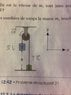# Second Newton's law in rotation with pulley.

• PhysicsKush
If it is making the pulley rotate more slowly, then the frictional force is opposing the motion of the pulley, and the equation for the torque is zero. If it is tending to make the pulley rotate faster, then the frictional force is not opposing the motion of the pulley, and the equation for the torque is I*(ay//r).f

## Homework Statement

The rope doesn't slide on the pulley, it's mass is negligible and it doesn't stretch .The pulley's moment of inertia is 0.00300 kg * m^2 with a radius of 10 cm. As the first mass goes down the rope's friction generates a moment of force of 0.150 N*m opposed to the angular speed of the pulley. Initially , both masses are motionless and m1 is at a height of 1.5m(h = 1.5m).
m1 = 1.5 kg
m2 = 0.8 kg

https://gyazo.com/074104f2af4ac27811136c21b4e1bcfb

## Homework Equations

τ = r*F
τresultant = Σ(τ) = I* αz
Fresultant = ma
αz = aθ / r
-ay1 = ay2

## The Attempt at a Solution

T1 = -m1ay1 + m1g
T2 = m2ay2 + m2g
-T1 + T2 - 1.5 = I*ay1
replacing the values in the last equation with the first two equations and isolating a results 3.69 m/s2

the real solution is a1 = -2.06 m/s2

#### Attachments

•ignore.jpg
29.9 KB · Views: 430
The equations used by you are valid for massless pulley. As it is given that pulley has MI, which means it has mass. So please use energy conservation to solve this problemM1 loses gravitational energy m2 gains and both m1, m2 gain translational KE and pulley gains rotational KE = 0.5*I*(v/r)^2. If magnitude of acceleartion is ay then angular acceleration of pulley = ay/r. Please give it a try.

If you wish to apply, then Tr = torque = I*(ay//r)

If you wish to apply, then Tr = torque = I*(ay//r)
yes ,but what about the friction ?

I don't understand the information given regarding frictional moment. We are told that the rope does not slip, we know the two suspended masses, and we know the moment of inertia of the pulley. Assuming this is in Earth's surface gravity, g, there is enough information to find the torque the rope exerts on the pulley. And it will not oppose the angular speed of the pulley.
The only way I can make sense of the problem is if it should have said that the axle exerts that frictional torque opposing the rotation of the pulley.
Assuming that is what the question said, check the signs in your equation for the pulley's acceleration. Is T1 tending to make the pulley rotate faster or more slowly?